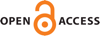Download this articleFor screen For printingRecent IssuesThe Journal About the Journal Editorial Board Editorial Interests Subscriptions Submission Guidelines Submission Page Policies for Authors Ethics Statement ISSN (electronic): 1472-2739 ISSN (print): 1472-2747 Author Index To Appear Other MSP JournalsProper $2$–equivalences between infinite ended finitely presented groups

### Manuel Cárdenas, Francisco Fernández Lasheras, Antonio Quintero and Ranja Roy

Algebraic & Geometric Topology 23 (2023) 1–11##### Abstract

Recall that two finitely presented groups $G$ and $H$ are “proper $2$–equivalent” if they can be realized by finite $2$–dimensional CW–complexes whose universal covers are proper $2$–equivalent as (strongly) locally finite CW–complexes. This purely topological relation is coarser than the quasi-isometry relation, and those groups which are $1$–ended and semistable at infinity are classified, up to proper $2$–equivalence, by their fundamental pro-group. We show that if $G$ and $H$ are proper $2$–equivalent and semistable at each end, then any two finite graph of groups decompositions of $G$ and $H$ with finite edge groups and finitely presented vertex groups with at most one end must have the same set of proper $2$–equivalence classes of (infinite) nonsimply connected at infinity vertex groups (without multiplicities). Moreover, those simply connected at infinity vertex groups in such a decomposition (if any) are all proper $2$–equivalent to $ℤ×ℤ×ℤ$. Thus, under the semistability hypothesis, this answers a question concerning the classification of infinite ended finitely presented groups up to proper $2$–equivalence, and shows again the behavior of proper $2$–equivalences versus quasi-isometries, in which the geometry of the group is taken into account.

##### Keywords
proper homotopy, proper $2$–equivalence, quasi-isometry, finitely presented group
Primary: 57M07
Secondary: 57M10
##### Publication